# Vapnik-Chervonenkis dimension

(diff) ← Older revision | Latest revision (diff) | Newer revision → (diff)

Vapnik–Červonenkis dimension, VC-dimension

Let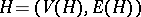be a hypergraph. The Vapnik–Chervonenkis dimension ofis the largest cardinality of a subsetof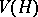that is scattered by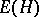, i.e. such that for allthere is anwith. Thus, it is the same as the index of a Vapnik–Chervonenkis class. It is usually denoted by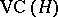.

Computing the Vapnik–Chervonenkis dimension is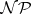-hard (cf. also) for many classes of hypergraphs, [a1], [a2].

The Vapnik–Chervonenkis dimension plays an important role in learning theory, especially in PAC learning (probably approximately correct learning). Thus, learnability of classes of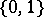-valued functions is equivalent to finiteness of the Vapnik–Chervonenkis dimension, [a3].

For the role of the Vapnik–Chervonenkis dimension in neural networks, see, e.g., [a4], [a5].

The independence number of a hypergraphis the maximal cardinality of a subsetofthat does not contain any(see also Graph, numerical characteristics of a). This notion is closely related with, [a6], [a7].

How to Cite This Entry:
Vapnik-Chervonenkis dimension. Encyclopedia of Mathematics. URL: http://encyclopediaofmath.org/index.php?title=Vapnik-Chervonenkis_dimension&oldid=11947
This article was adapted from an original article by M. Hazewinkel (originator), which appeared in Encyclopedia of Mathematics - ISBN 1402006098. See original article